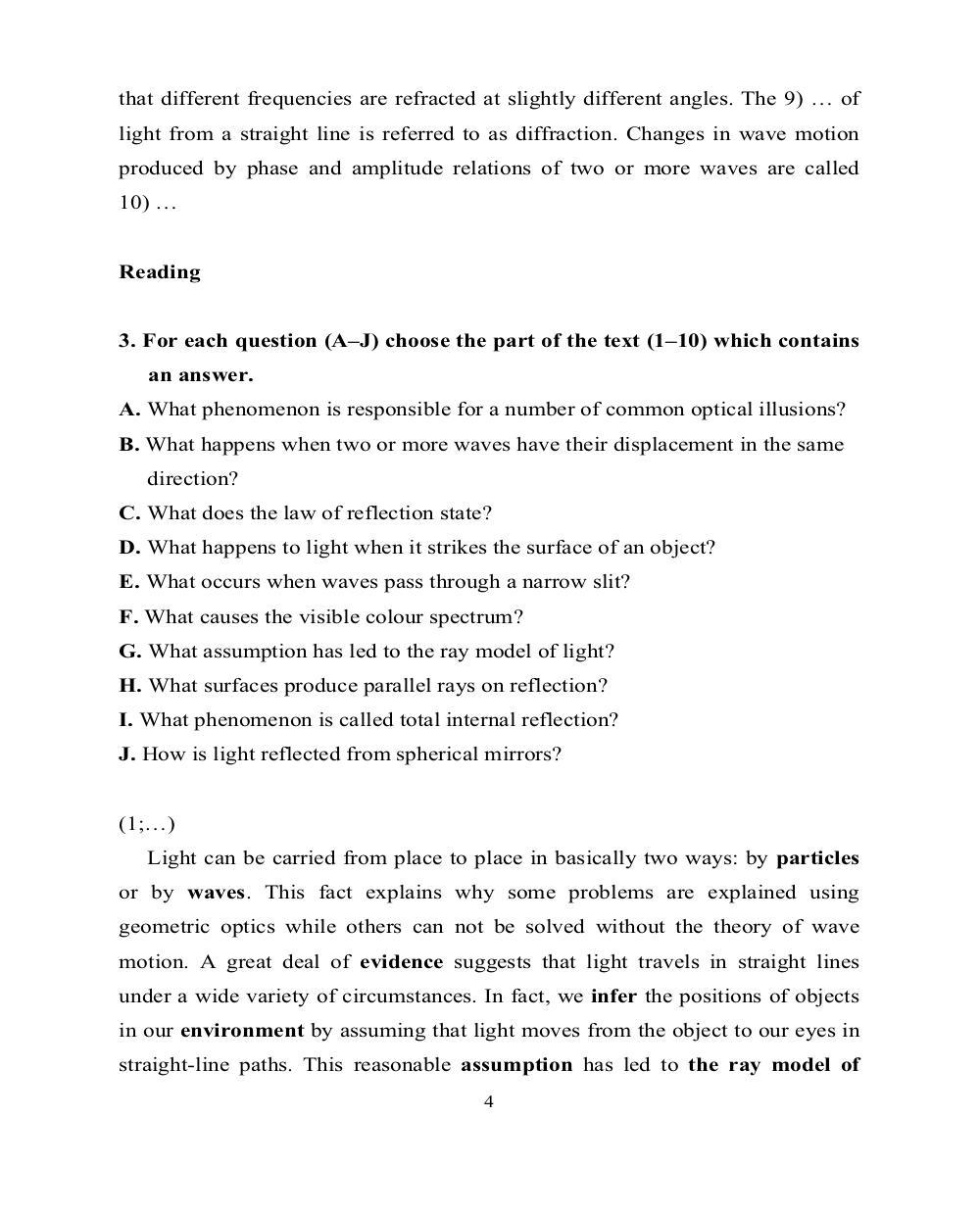# Английский язык. Ч.3 (Units 9,10). Ильичева Н.А - 4 стр.

Составители:

Рубрика:

• ## Иностранный язык

that different frequencies are refracted at slightly different angles. The 9) … of
light from a straight line is referred to as diffraction. Changes in wave motion
produced by phase and amplitude relations of two or more waves are called
10) …
3. For each question (A–J) choose the part of the text (1–10) which contains
A. What phenomenon is responsible for a number of common optical illusions?
B. What happens when two or more waves have their displacement in the same
direction?
C. What does the law of reflection state?
D. What happens to light when it strikes the surface of an object?
E. What occurs when waves pass through a narrow slit?
F. What causes the visible colour spectrum?
G. What assumption has led to the ray model of light?
H. What surfaces produce parallel rays on reflection?
I. What phenomenon is called total internal reflection?
J. How is light reflected from spherical mirrors?
(1;…)
Light can be carried from place to place in basically two ways: by particles
or by waves. This fact explains why some problems are explained using
geometric optics while others can not be solved without the theory of wave
motion. A great deal of evidence suggests that light travels in straight lines
under a wide variety of circumstances. In fact, we infer the positions of objects
in our environment by assuming that light moves from the object to our eyes in
straight-line paths. This reasonable assumption has led to the ray model of
4that different frequencies are refracted at slightly different angles. The 9) … of
light from a straight line is referred to as diffraction. Changes in wave motion
produced by phase and amplitude relations of two or more waves are called
10) …

3. For each question (A–J) choose the part of the text (1–10) which contains
A. What phenomenon is responsible for a number of common optical illusions?
B. What happens when two or more waves have their displacement in the same
direction?
C. What does the law of reflection state?
D. What happens to light when it strikes the surface of an object?
E. What occurs when waves pass through a narrow slit?
F. What causes the visible colour spectrum?
G. What assumption has led to the ray model of light?
H. What surfaces produce parallel rays on reflection?
I. What phenomenon is called total internal reflection?
J. How is light reflected from spherical mirrors?

(1;…)
Light can be carried from place to place in basically two ways: by particles
or by waves. This fact explains why some problems are explained using
geometric optics while others can not be solved without the theory of wave
motion. A great deal of evidence suggests that light travels in straight lines
under a wide variety of circumstances. In fact, we infer the positions of objects
in our environment by assuming that light moves from the object to our eyes in
straight-line paths. This reasonable assumption has led to the ray model of
4